# NCERT Exemplar Class 8 Science Solutions for Chapter 11 - Force and Pressure

## NCERT Exemplar Solutions Class 8 Science Chapter 11 – Free PDF Download

The NCERT Exemplar for Class 8 Science Chapter 11 Force and Pressure explains the topics mentioned in CBSE Class 8 Chapter 11. By studying this Exemplar thoroughly, students are able to strengthen their exam preparation. This will help them determine their strengths and weaknesses. NCERT Exemplar Problems provided here are not meant to provide you only with a question bank for examinations, but are primarily meant to enhance your learning process. Practising Exemplar will help you solve different kinds of questions and numericals, which are important from the examination point of view.

Force and Pressure is one of the most important chapters of CBSE Class 8 Science. In this chapter, students will learn about the basic concepts of force and pressure. To understand the concepts of force and pressure and to score good marks in the exam, students must understand the basics of the chapter and solve the important questions. To help students, we have provided the NCERT Exemplar for Class 8 Science Chapter 11 Force and Pressure.

### Download the PDF of the NCERT Exemplar Class 8 Science Solutions for Chapter 11 – Force and Pressure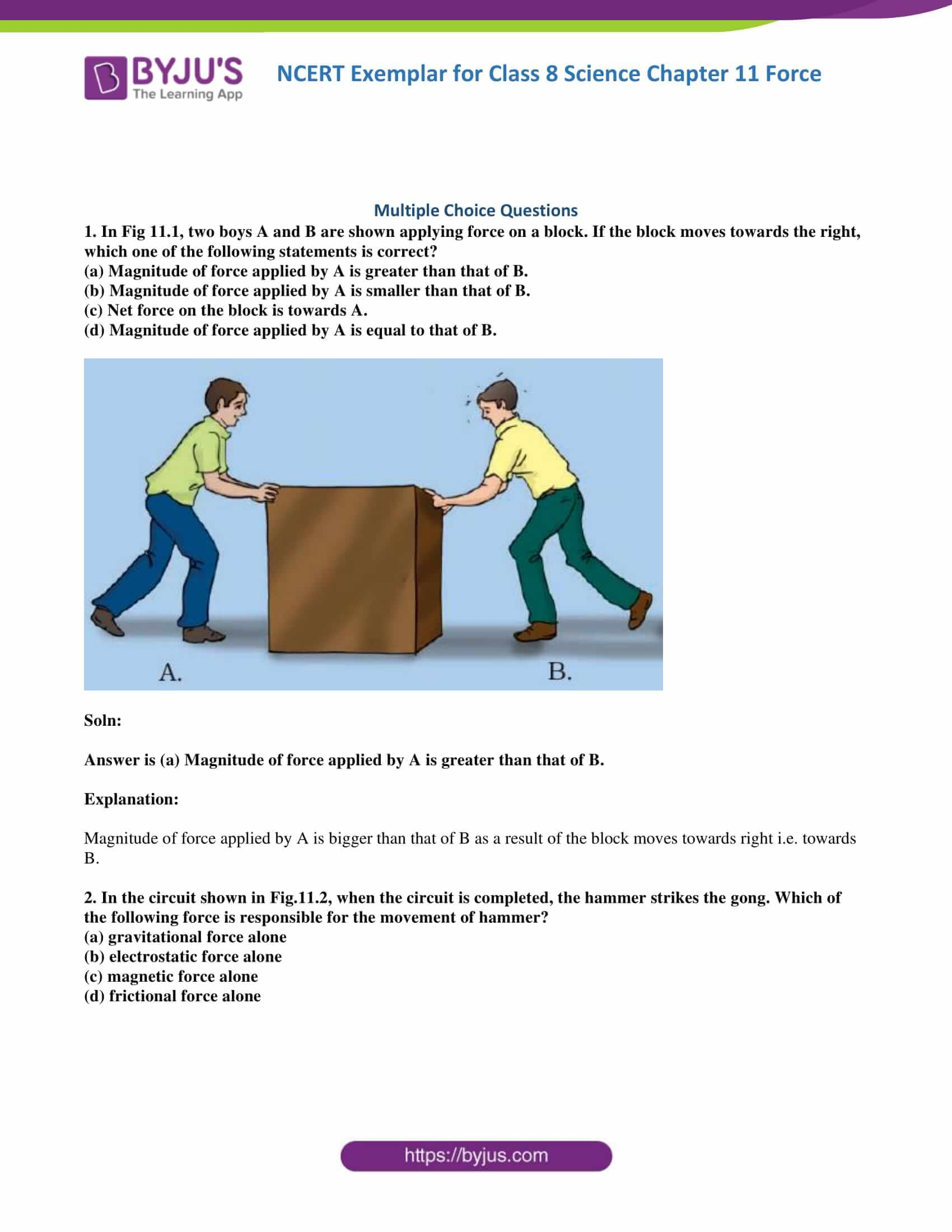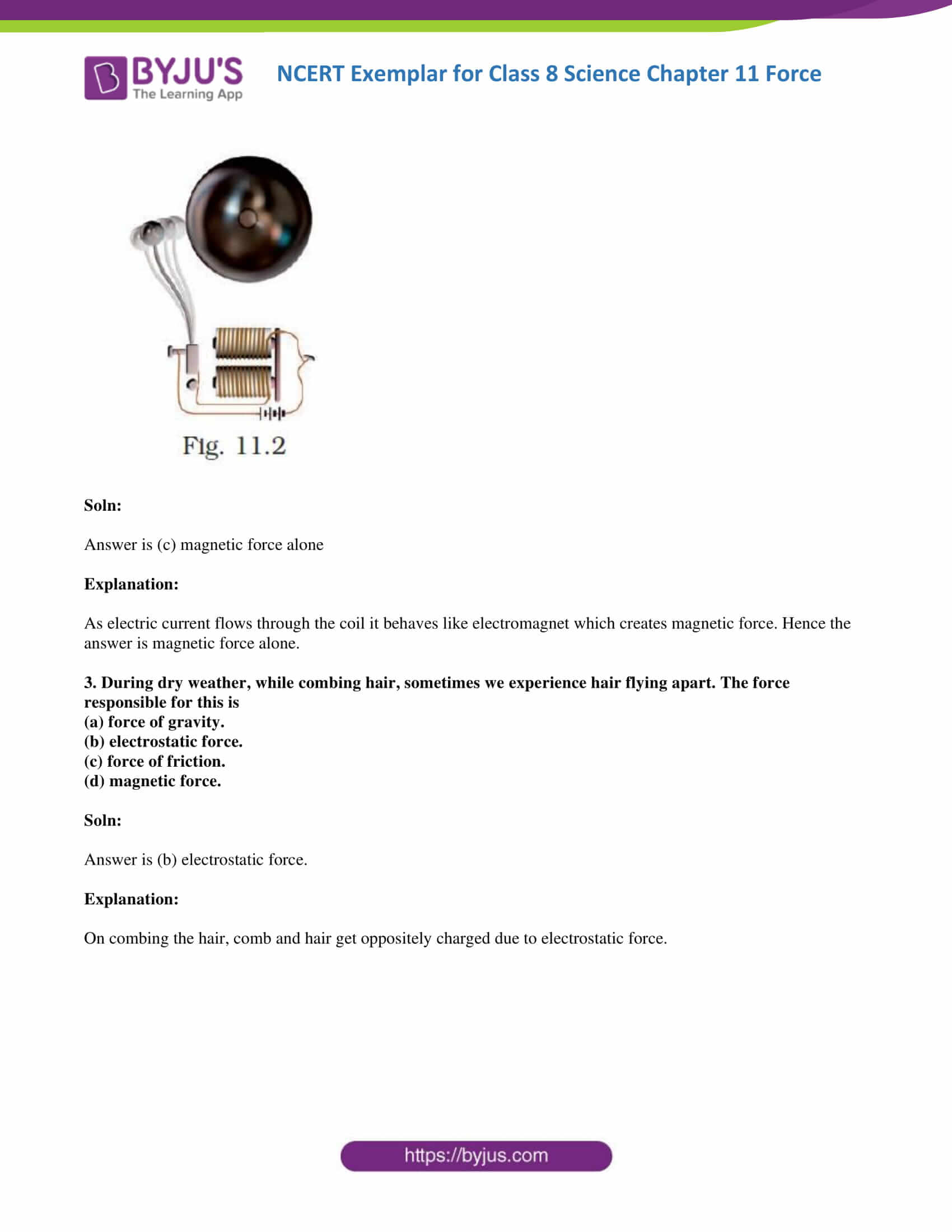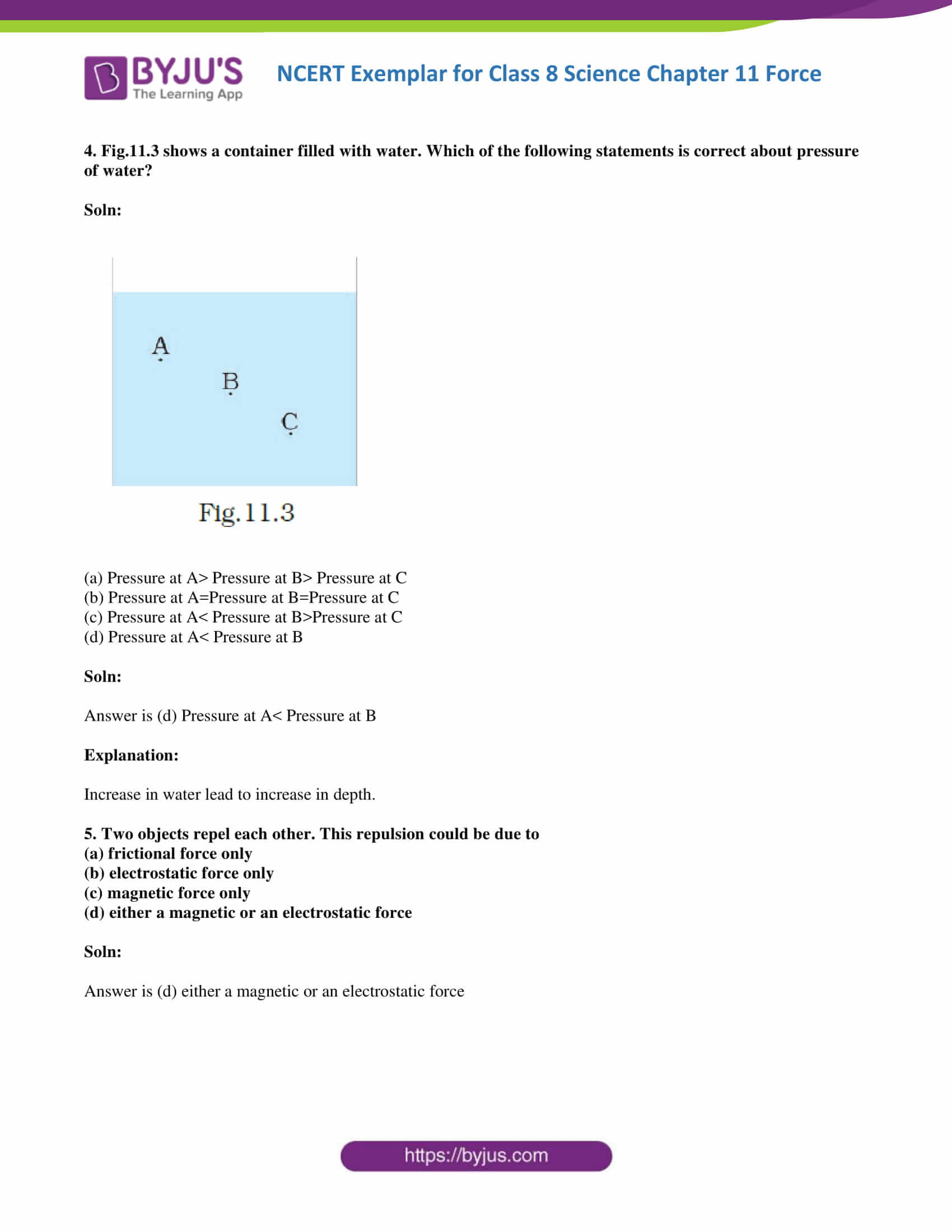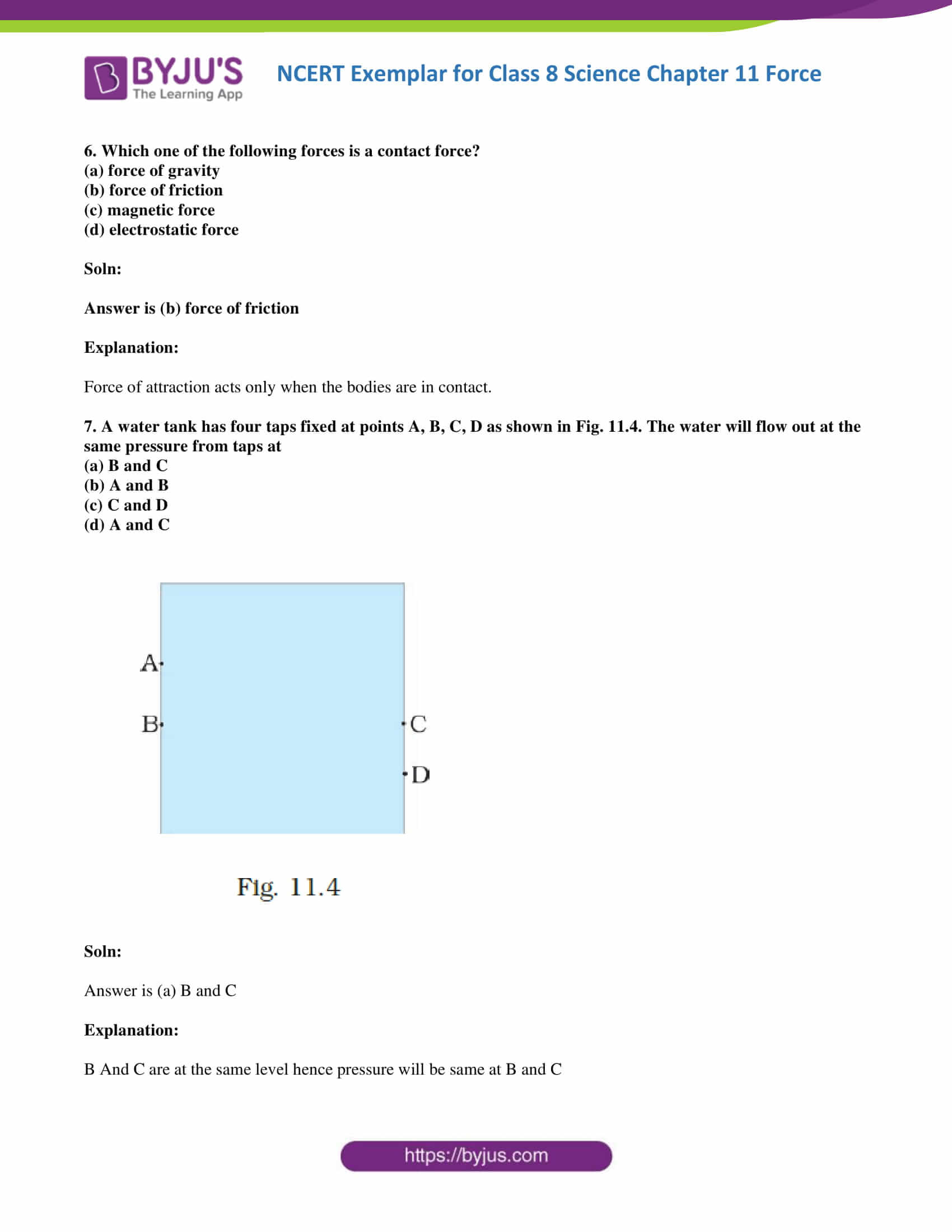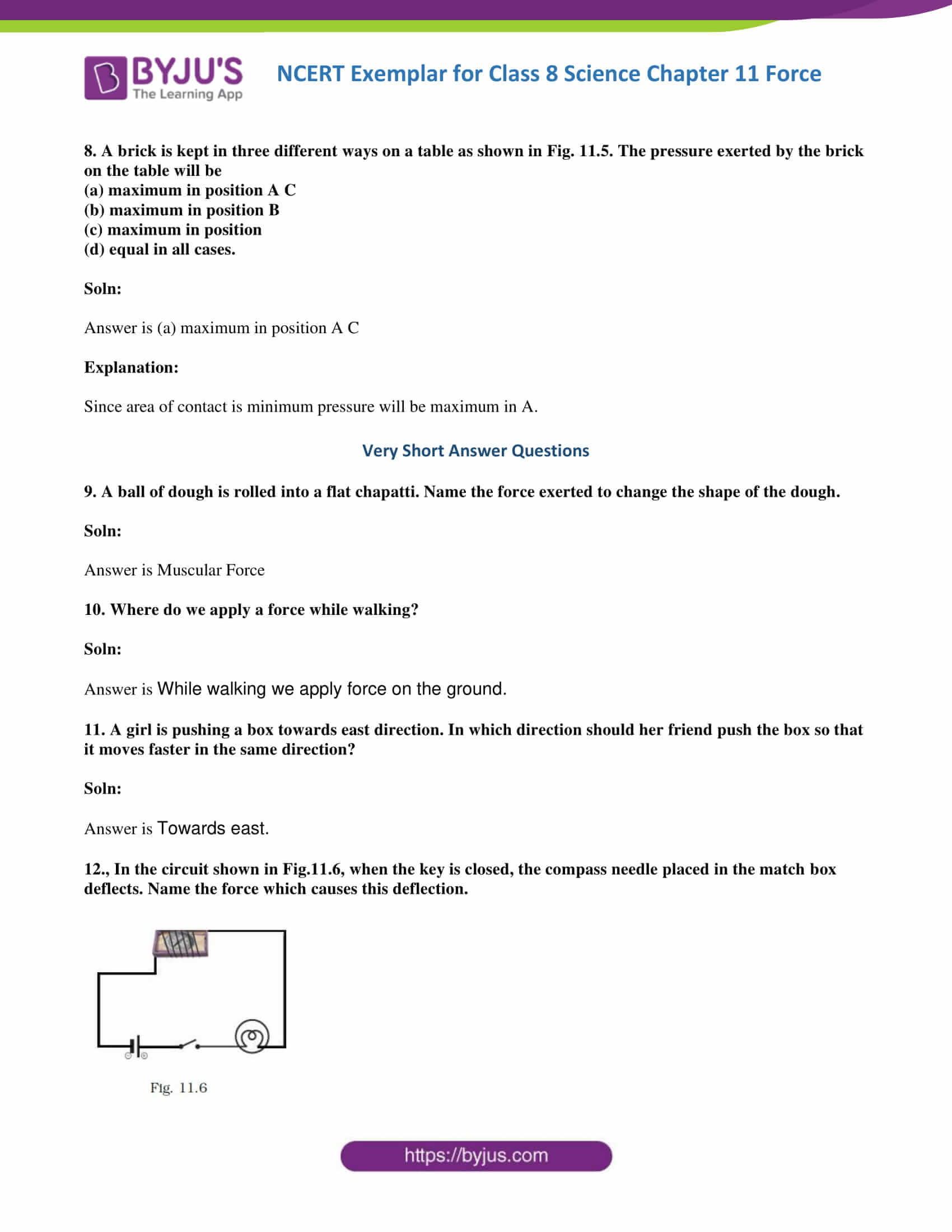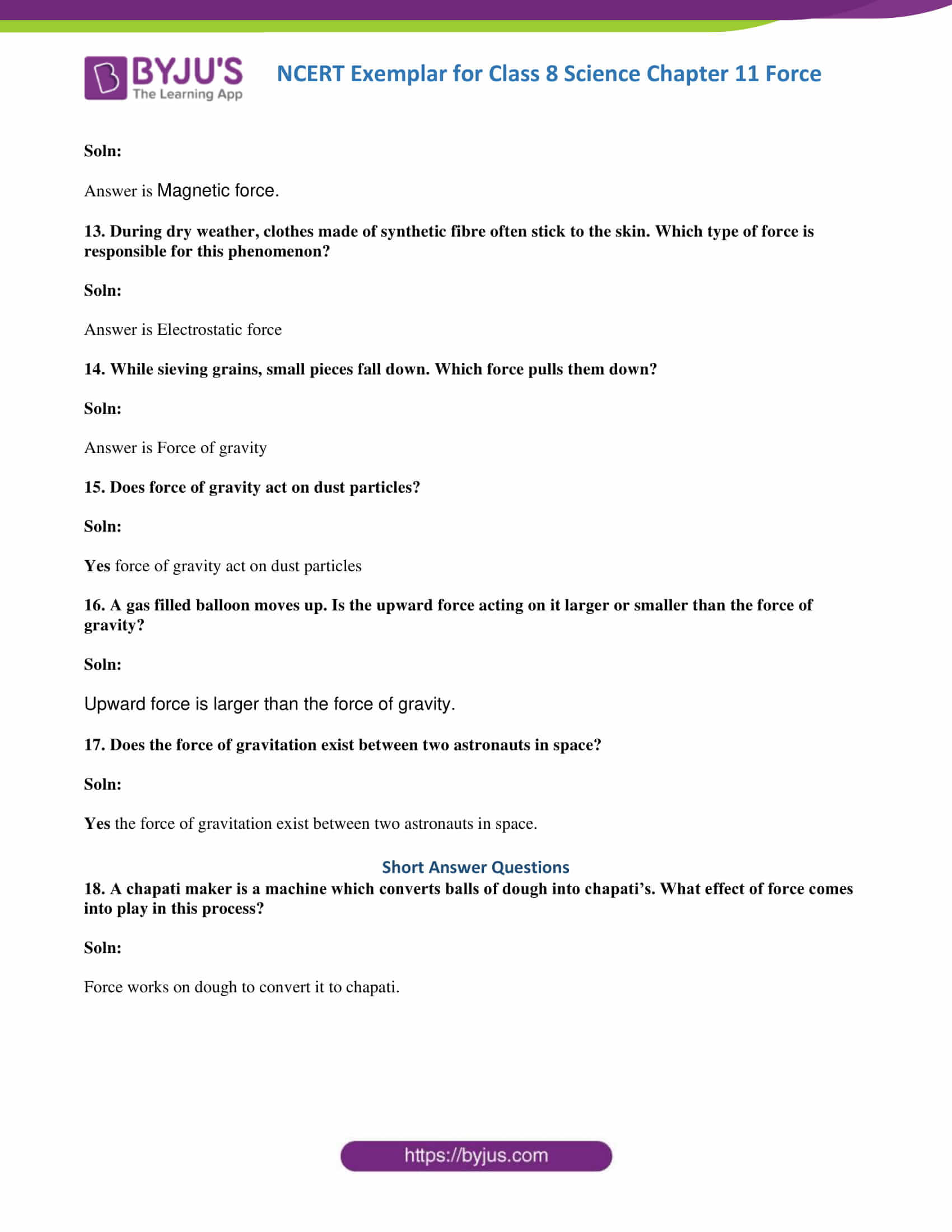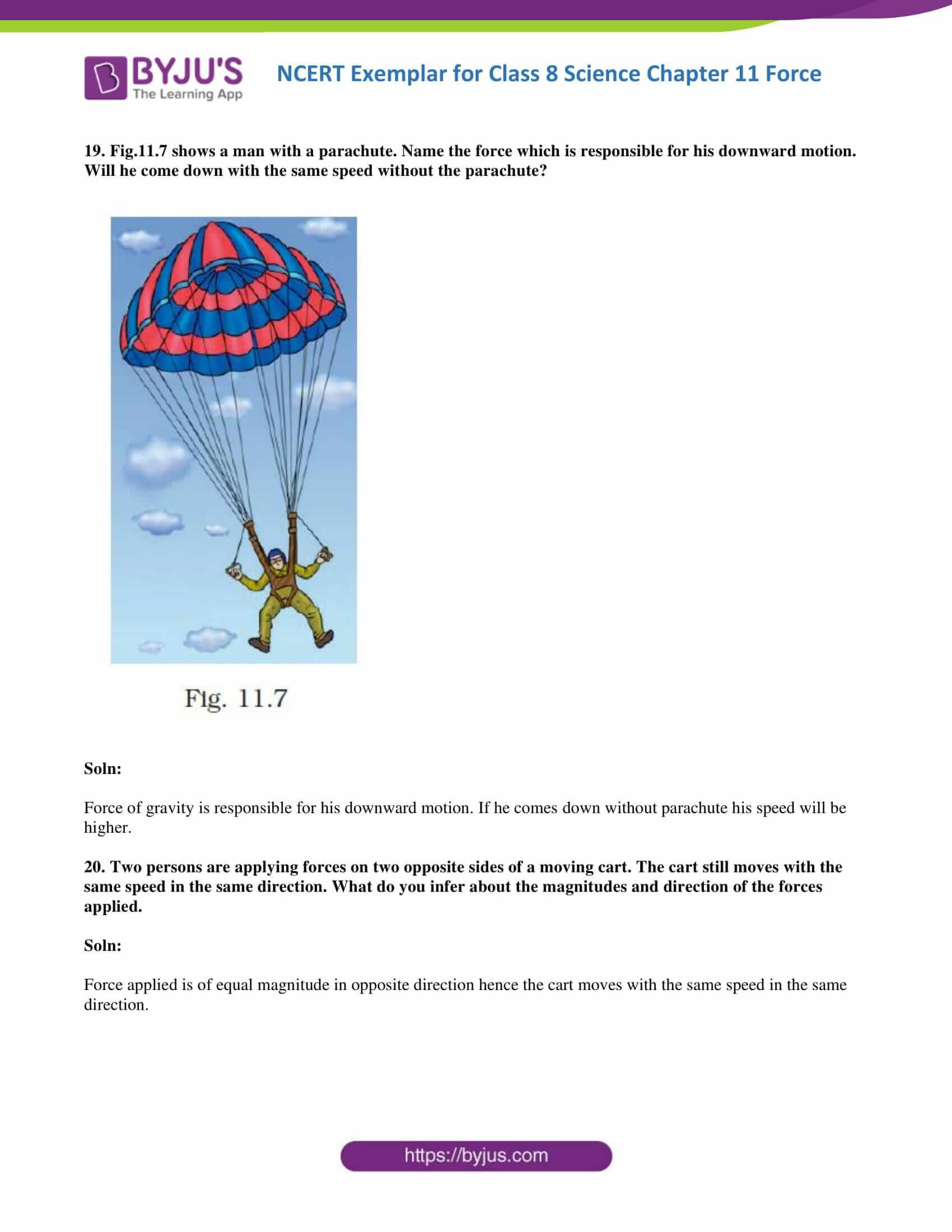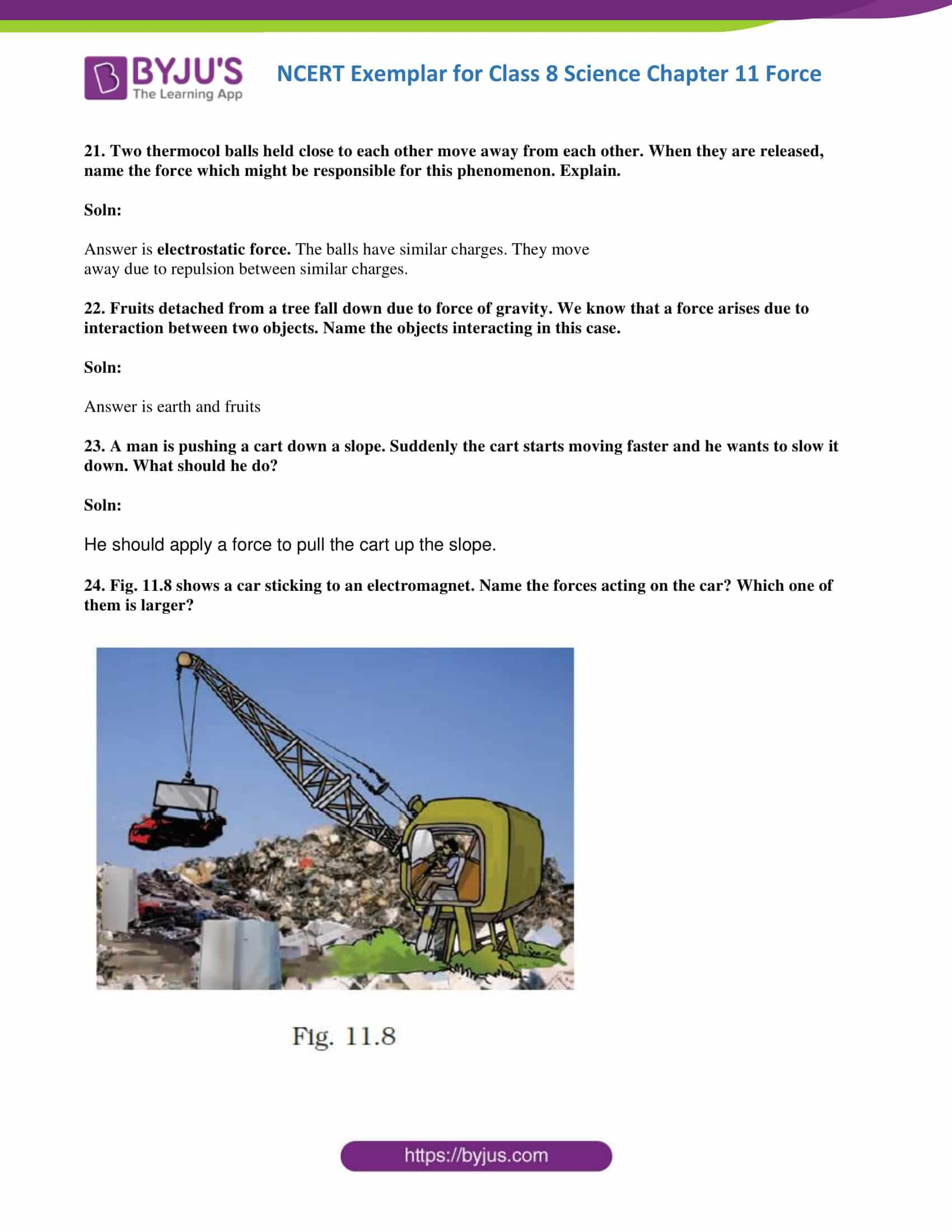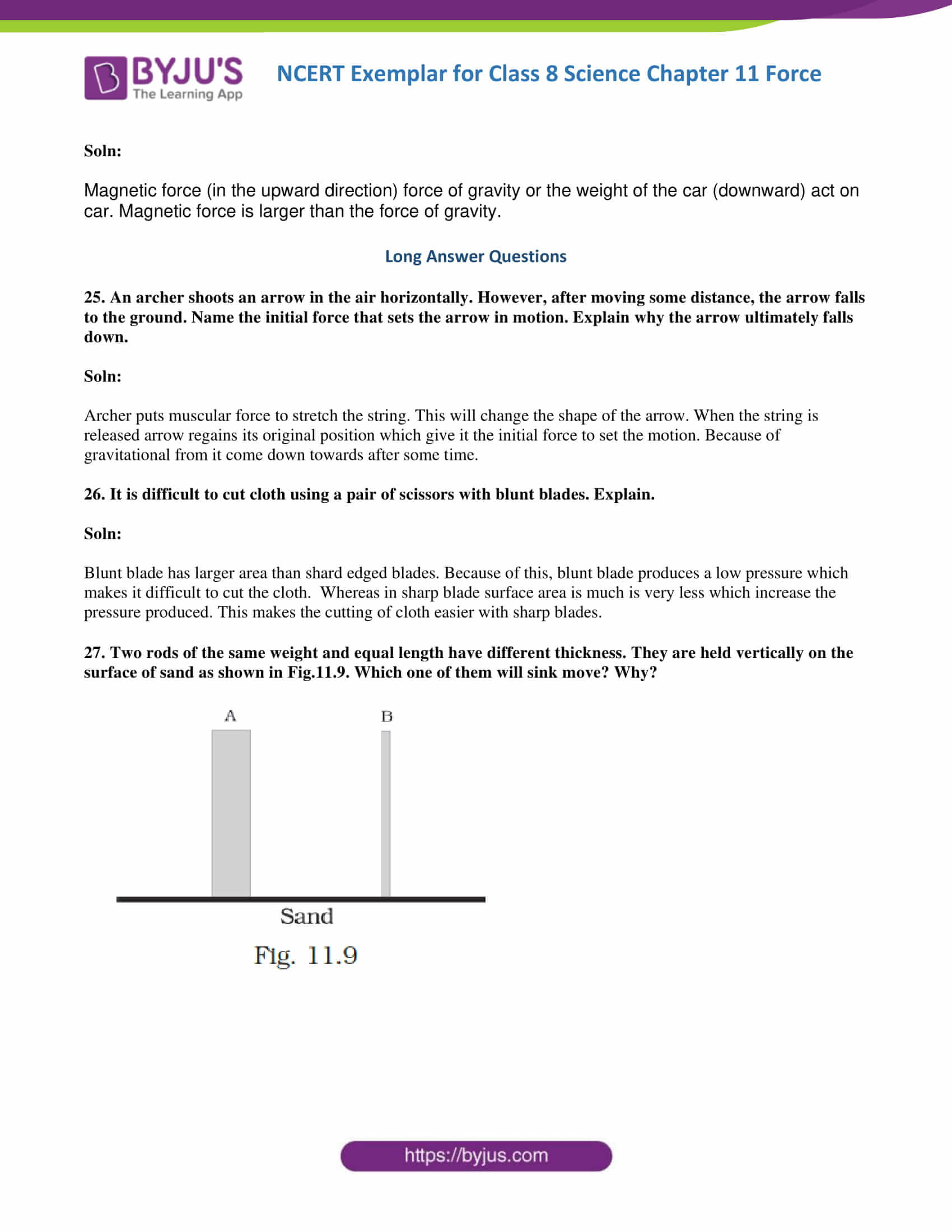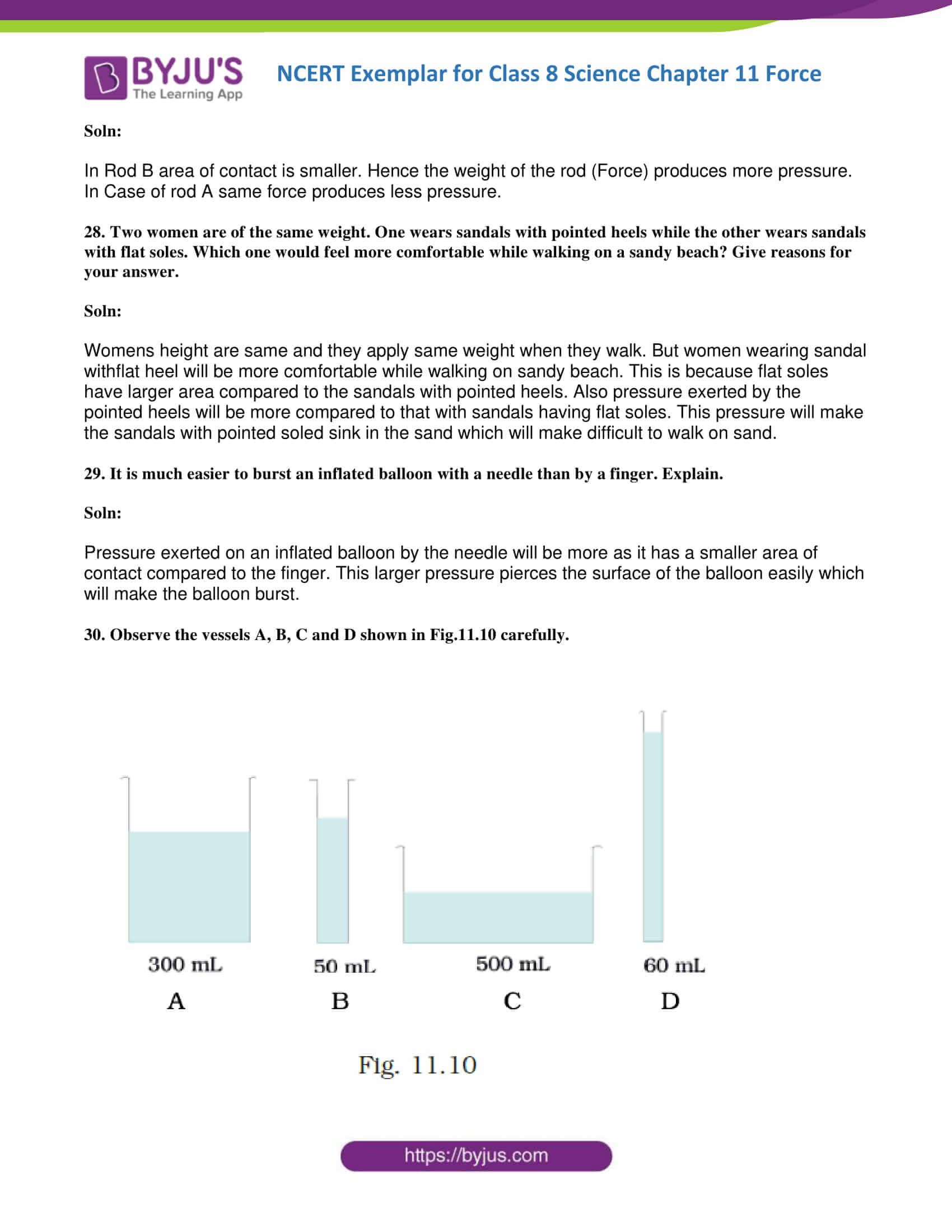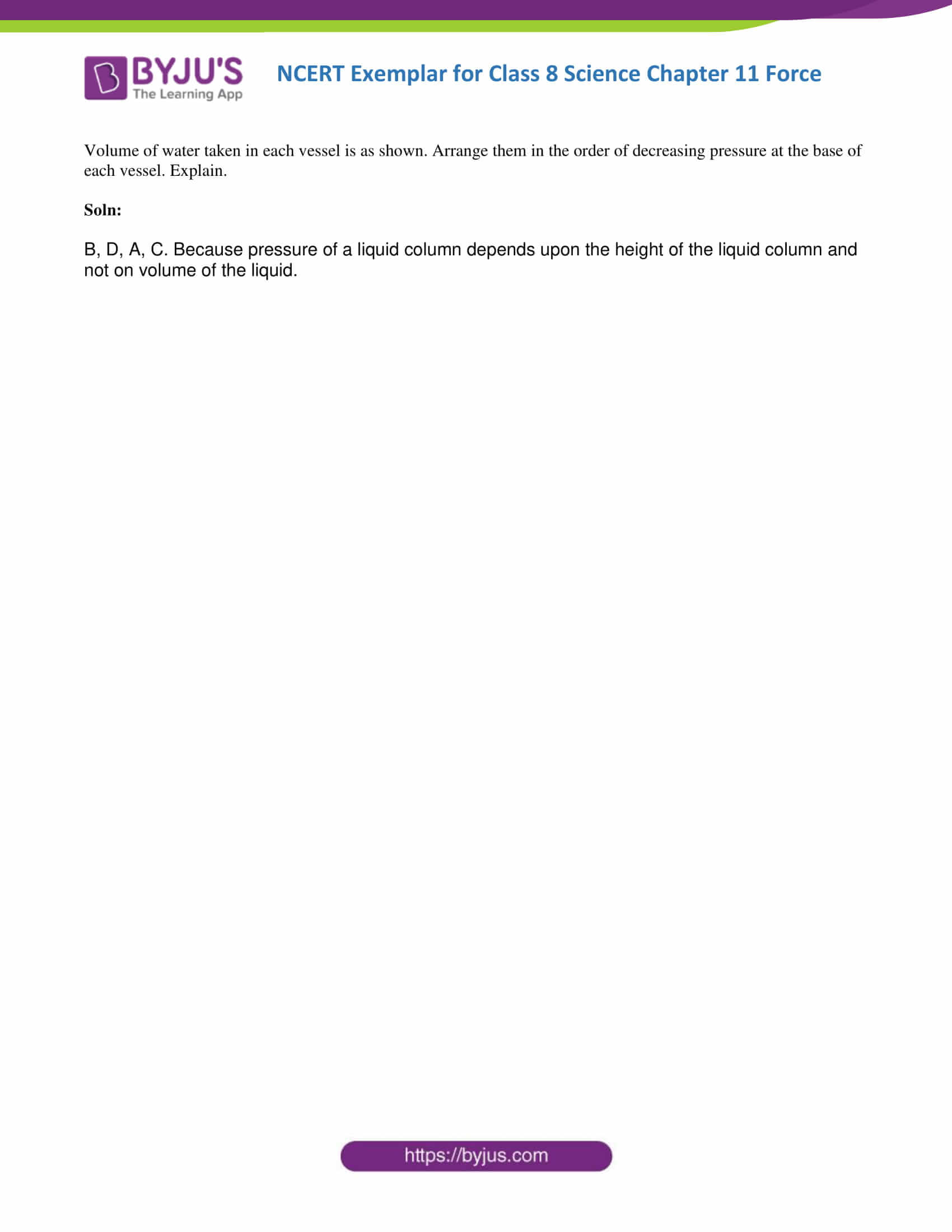### Multiple Choice Questions

1. In Fig 11.1, two boys A and B are shown applying force on a block. If the block moves towards the right, which one of the following statements is correct?

(a) The magnitude of force applied by A is greater than that of B.

(b) The magnitude of force applied by A is smaller than that of B.

(c) The net force on the block is towards A.

(d) The magnitude of force applied by A is equal to that of B.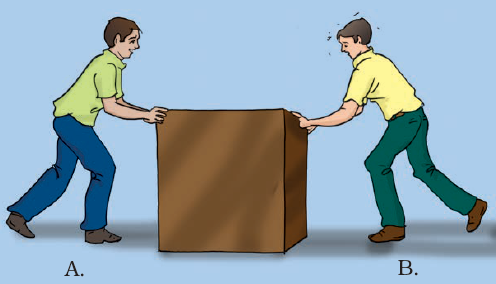Soln:

Answer is (a) Magnitude of force applied by A is greater than that of B.

Explanation:

The magnitude of force applied by A is bigger than that of B as a result of the block moves towards right i.e. towards B.

2. In the circuit shown in Fig.11.2, when the circuit is completed, the hammer strikes the gong. Which of the following force is responsible for the movement of the hammer?

(a) gravitational force alone

(b) electrostatic force alone

(c) magnetic force alone

(d) frictional force alone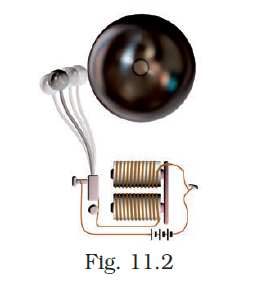Soln:

Answer is (c) magnetic force alone

Explanation:

As electric current flows through the coil it behaves like an electromagnet which creates magnetic force. Hence the answer is magnetic force alone.

3. During dry weather, while combing hair, sometimes we experience hair flying apart. The force responsible for this is

(a) force of gravity.

(b) electrostatic force.

(c) the force of friction.

(d) magnetic force.

Soln:

The answer is (b) electrostatic force.

Explanation:

On combing the hair, comb and hair get oppositely charged due to electrostatic force.

4. Fig.11.3 shows a container filled with water. Which of the following statements is correct about the pressure of water?

Soln: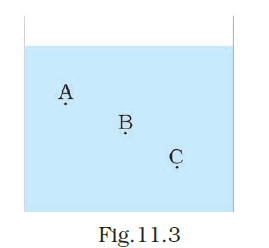(a) The pressure at A > Pressure at B > Pressure at C.

(b) The pressure at A = Pressure at B = Pressure at C.

(c) The pressure at A <  Pressure at B > Pressure at C.

(d) The pressure at A <  Pressure at B.

Soln:

The answer is (d) Pressure at A < Pressure at B

Explanation:

Increase in water leads to an increase in depth.

5. Two objects repel each other. This repulsion could be due to

(a) frictional force only

(b) electrostatic force only

(c) magnetic force only

(d) either a magnetic or an electrostatic force

Soln:

The answer is (d) either a magnetic or an electrostatic force

Explanation: when two objects are experiencing repulsive force because there may be an electrostatic force or a magnetic force.

6. Which one of the following forces is a contact force?

(a) force of gravity

(b) force of friction

(c) magnetic force

(d) electrostatic force

Soln:

The answer is (b) force of friction

Explanation:

Force of attraction acts only when the bodies are in contact.

7. A water tank has four taps fixed at points A, B, C, D as shown in Fig. 11.4. The water will flow out at the same pressure from taps at

(a) B and C

(b) A and B

(c) C and D

(d) A and C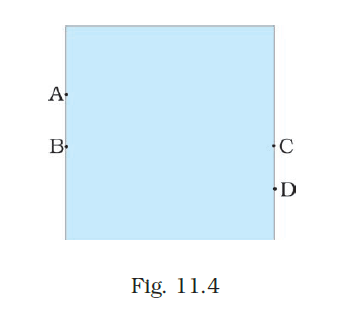Soln:

Answer is (a) B and C

Explanation:

B and C are at the same level, hence pressure will be the same at B and C.

8. A brick is kept in three different ways on a table as shown in Fig. 11.5. The pressure exerted by the brick on the table will be

(a) maximum in position A-C

(b) maximum in position B

(c) maximum in position

(d) equal in all cases.

Soln:

Answer is (a) maximum in position A-C

Explanation:

Since the area of contact is minimum pressure will be maximum in A.

9. A ball of dough is rolled into a flat chapatti. Name the force exerted to change the shape of the dough.

Soln:

Muscular Force.

10. Where do we apply a force while walking?

Soln:

While walking we apply force on the ground.

11. A girl is pushing a box towards the east direction. In which direction should her friend push the box so that it moves faster in the same direction?

Soln:

Towards the east.

12., In the circuit shown in Fig.11.6, when the key is closed, the compass needle placed in the matchbox deflects. Name the force which causes this deflection.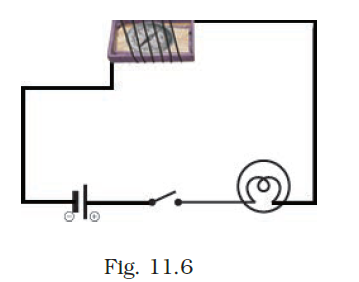Soln:

13. During dry weather, clothes made of synthetic fibre often stick to the skin. Which type of force is responsible for this phenomenon?

Soln:

14. While sieving grains, small pieces fall down. Which force pulls them down?

Soln:

Force of gravity.

15. Does the force of gravity act on dust particles?

Soln:

Yes, the force of gravity act on dust particles.

16. A gas-filled balloon moves up. Is the upward force acting on it larger or smaller than the force of gravity?

Soln:

The upward force is larger than the force of gravity.

17. Does the force of gravitation exist between two astronauts in space?

Soln:

Yes, the force of gravitation exists between two astronauts in space.

18. A chapati maker is a machine which converts balls of dough into chapati. What effect of force comes into play in this process?

Soln:

Force works on the dough to convert it to chapati.

19. Fig.11.7 shows a man with a parachute. Name the force which is responsible for his downward motion. Will he come down with the same speed without the parachute?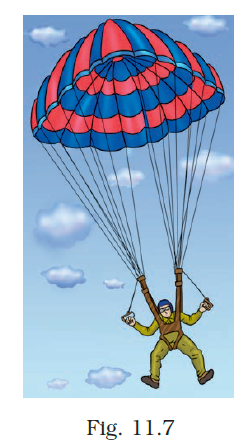Soln:

Force of gravity is responsible for his downward motion. If he comes down without parachute his speed will be higher.

20. Two persons are applying forces on two opposite sides of a moving cart. The cart still moves with the same speed in the same direction. What do you infer about the magnitudes and direction of the forces applied?

Soln:

Force applied is of equal magnitude in the opposite direction hence the cart moves with the same speed in the same direction.

21. Two thermocol balls held close to each other move away from each other. When they are released, name the force which might be responsible for this phenomenon. Explain.

Soln:

Two Thermocol balls held close to each other move away from each other, which is because of electrostatic force. The balls having similar charges move away due to repulsion between similar charges.

22. Fruits detached from a tree fall down due to force of gravity. We know that a force arises due to the interaction between two objects. Name the objects interacting in this case.

Soln:

Earth and fruits.

23. A man is pushing a cart down a slope. Suddenly the cart starts moving faster and he wants to slow it down. What should he do?

Soln:

He should apply a force to pull the cart up the slope.

24. Fig. 11.8 shows a car sticking to an electromagnet. Name the forces acting on the car? Which one of them is larger?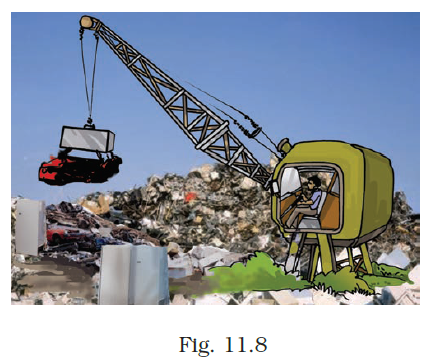Soln:

Magnetic force (in the upward direction) force of gravity or the weight of the car (downward) act on car. Magnetic force is larger than the force of gravity.

25. An archer shoots an arrow in the air horizontally. However, after moving some distance, the arrow falls to the ground. Name the initial force that sets the arrow in motion. Explain why the arrow ultimately falls down.

Soln:

Archer puts muscular force to stretch the string. This will change the shape of the arrow. When the string is released arrow regains its original position which gives it the initial force to set the motion. Because of gravitational from it comes down towards after some time.

26. It is difficult to cut cloth using a pair of scissors with blunt blades. Explain.

Soln:

The blunt blade has a larger area than shard edged blades. Because of this, blunt blade produces a low pressure which makes it difficult to cut the cloth. Whereas in sharp blade surface area is much is very less which increase the pressure produced. This makes the cutting of cloth easier with sharp blades.

27. Two rods of the same weight and equal length have different thickness. They are held vertically on the surface of sand as shown in Fig.11.9. Which one of them will sink move? Why?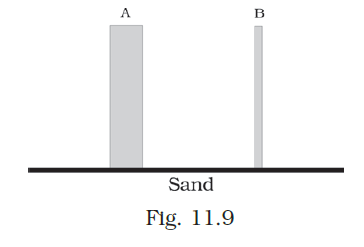Soln:

In Rod B area of contact is smaller. Hence the weight of the rod (Force) produces more pressure. In Case of the rod, the same force produces less pressure.

28. Two women are of the same weight. One wears sandals with pointed heels while the other wears sandals with flat soles. Which one would feel more comfortable while walking on a sandy beach? Give reasons for your answer.

Soln:

Women’s height are same and they apply the same weight when they walk. But women wearing sandal with a flat heel will be more comfortable while walking on a sandy beach. This is because flat soles

have larger area compared to the sandals with pointed heels. Also, the pressure exerted by the

pointed heels will be more compared to that with sandals having flat soles. This pressure will make the sandals with pointed soled sink in the sand which will make difficult to walk on sand.

29. It is much easier to burst an inflated balloon with a needle than by a finger. Explain.

Soln:

The pressure exerted on an inflated balloon by the needle will be more as it has a smaller area of contact compared to the finger. This larger pressure pierces the surface of the balloon easily which will make the balloon burst.

30. Observe the vessels A, B, C and D shown in Fig.11.10 carefully.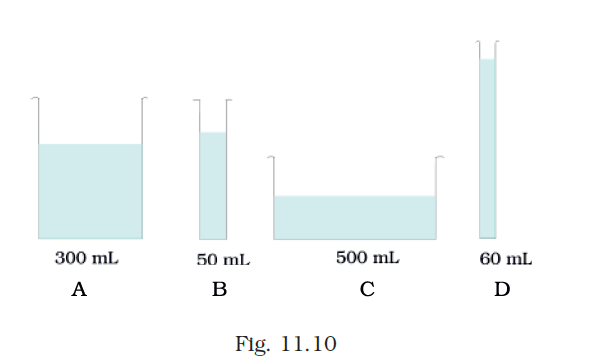The volume of water taken in each vessel is as shown. Arrange them in the order of decreasing pressure at the base of each vessel. Explain.

Soln:

B, D, A, C. Because the pressure of a liquid column depends upon the height of the liquid column and not on volume of the liquid.

### Sub-topics of the CBSE syllabus Class 8 Science Chapter 11 Force and Pressure

1. Force – A Push or Pull
2. Forces Are Due to an Interaction
3. Exploring Forces
4. A Force Can Change the State of Motion
5. Force Can Change the Shape of an Object
6. Contact Forces
7. Non-contact Forces
8. Pressure
9. Pressure Exerted by Liquids and Gases
10. Atmospheric Pressure

BYJU’S presents outstanding NCERT Solutions, study materials, sample papers, previous years’ question papers and video and animation lessons for a thorough understanding and to memorise topics for a longer period. To get access to all study materials we provide, log on to BYJU’S website or download BYJU’S – The Learning App.

## Frequently Asked Questions on NCERT Exemplar Solutions for Class 8 Science Chapter 11

Q1

### What are the topics covered under Chapter 11 of NCERT Exemplar Solutions for Class 8 Science?

The topics covered under Chapter 11 of NCERT Exemplar Solutions for Class 8 Science are as listed
1. Force – A Push or Pull
2. Forces Are Due to an Interaction
3. Exploring Forces
4. A Force Can Change the State of Motion
5. Force Can Change the Shape of an Object
6. Contact Forces
7. Non-contact Forces
8. Pressure
9. Pressure Exerted by Liquids and Gases
10. Atmospheric Pressure
Q2

### What are non-contact forces in Chapter 11 of NCERT Exemplar Solutions for Class 8 Science?

Forces that act through spaces without making direct contact with the body are called non-contact forces. Examples of non-contact forces are gravitational force, electrostatic force and magnetic force. The force exerted by a magnet on other magnets is called magnetic force. Magnetic force and electrostatic force act on an object from a distance, and that’s the reason they are non-contact forces. The strength of gravity is an attractive force that is exerted by the Earth on objects, which makes them fall to the land. The weight of a body is the force that is pulled by the earth towards the centre. This concept can be clearly understood by students using the NCERT Exemplar Solutions created by the expert faculty at BYJU’S. The solutions contain explanations provided in a comprehensive manner to boost the exam preparation of students.
Q3

### Can students rely on NCERT Exemplar Solutions for Class 8 Science Chapter 11 from BYJU’S?

BYJU’S NCERT Exemplar Solutions for Class 8 Science Chapter 11 are created to assist students in learning key ideas. Every element is meticulously described to improve students’ conceptual understanding. The solutions also include a variety of shortcut approaches to effectively remember the principles. While answering textbook questions, students can refer to the solutions and find the best way of answering them without difficulty.